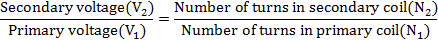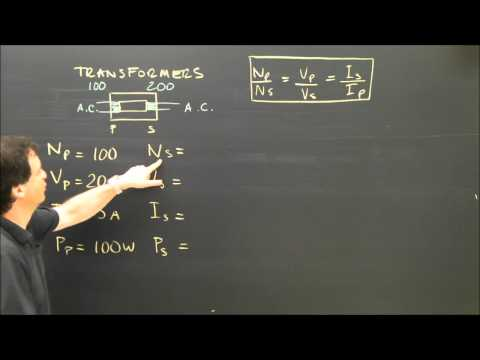## Transformer

Subject: Science

#### Overview

A transformer is a device used to convert low a.c. voltage into high a.c. voltage and vice versa. This note has short description about electric transformer and its type
##### Transformer

A transformer is a device used to convert low a.c. voltage into high a.c. voltage and vice versa. It is of two types: -

1. Step-up transformer
2. Step-down transformer

A step-up transformer converts low a.c. voltage into high a.c voltage and step-down transformer converts high a.c. voltage into low a.c voltage. The instrument is based on the principle of mutual induction.

Structure: It consists of a rectangular soft iron core made up of laminated sheets. There are two coils which are not connected to one another in any way. These coils are wound on the iron core. One of the coils may be connected to a source of a.c. This coil is called primary coil and the other is called secondary coil.The e.m.f. of a.c. source applied across the primary coil is called input voltage and e.m.f. induced across the secondary coil is called output voltage.

Principle: When an alternating e.m.f. is applied to the primary coil, a changing current flowing in it produces an alternating magnetic flux in it. This causes to change the magnetic flux linked with the secondary coil. An alternating e.m.f. is then induced in the secondary coil. It is called principle of mutual induction on which transformers are based.

The formula showing the relation between the primary voltage and secondary voltage is as follows: -#### Uses

1. Transformers are used in voltage regulators for computer, television, air conditioner, record player, etc.
2. It is used to transmit the alternating current over long distances.
3. It is used for doorbells, welding purpose and in electrical furnaces.
4. It can be used to prevent DC from passing from one circuit tothe other.
5. It can isolate two circuits electrically.
##### Things to remember
• A transformer is a device used to convert low a.c. voltage into high a.c. voltage and vice versa.
• Transformer is of two types: step-up transformer and step-down transformer.
• A solenoid behaves like a magnet during the flow of electric current through it.
• Electric bell is a source of sound that converts electrical energy into kinetic energy and then to sound energy.
• It includes every relationship which established among the people.
• There can be more than one community in a society. Community smaller than society.
• It is a network of social relationships which cannot see or touched.
• common interests and common objectives are not necessary for society.
##### Videos for Transformer##### Electrical Transformer Calculations Physics Tutorial
No, the transformer can"t change the electromotive force (e.m.f.) of a dry cell.

Transformer is needed during the both cases, either to increase the voltage or to decrease it. Without transformer no change in the voltage of alternating current can be made. That's why the use of alternating current is limited without transformer.

The core of the transformer is laminated just to reduce the loss in input current. When electric current flows in the core of a transformer, the internal current i.e. eddy current (which flows in a circular way) is set up in the body of core. This current has heating effect on the core. The un-laminated core acts as a single block of iron which is heated due to high amount of eddy current. If the core is laminated, the amount of eddy current is highly reduced. Therefore, the core of the transformer is laminated to avoid the heating of a transformer.
• Input current: The current that is given to the transformer is called input current.
• Output current: The current that is taken out from the transformer is called output current.
• Primary voltage: The voltage that appears across the primary coil of the transformer is called primary voltage.
• Secondary voltage: The voltage or potential difference that appears across the secondary coil of the transformer is called secondary voltage.
Any two differences between step-up transformer and step-down transformer are as follows: -
 Step-up transformer Step-down transformer It increases the output electricity more than input electricity. It decreases the output electricity more than input electricity. It increases the magnitude of AC emf. It decreases the magnitude of AC emf.

Solution,
Here,
Power of a bulb (P) = 100 W
Potential difference (V) = 220 V
Current electricity (I) = 5 Ampere
According to the formula,
P = V × I
or,100 × X = 220 × 5
or, X=or, X=or, X = 11
Hence 10 bulbs can be safely used in the circuit.
Solution,
Here,
Primary voltage (V1) = 220V
Turns in primary coil (N1) = 770
Secondary voltage (V2) = 120V
Turns in secondary coil (N2) = ?
Now, Using formula,420
he number of turns in the secondary coil is 420.
The transformer is used in alternating current either to increase the low voltage to high voltage to low voltage according to the required voltage.